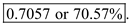# Quiz 6: The Link Between Operations and Finance

Business

There is no answer for this question

The Return-On-Invested Capital (ROIC) for a capital investment scenario can be defined as the following expression:Where, the 'return' is to be computed by deducting all the expenses from the sales for a given period which is generally taken as one year. a. Use Little's law to compute the flow rate where the Work-In-Process (WIP) and Flow time is known. In this case, the WIP is the number of seats available which is 50. The flow time is the overall time spent per customer in the restaurant. This includes the 50 minutes of waiting, food serving, eating, and checking-out time plus the 10 minutes of cleaning activity i.e. a total of 60 minutes. So, from the Little's law:So,Since the working time for the restaurant in a typical evening is 4 hours, the number of customers served in an evening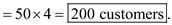b. Compute the sales per evening by multiplying the number of customers served per evening by the average payment by a customer. So,The cost per evening includes the wages, material cost, rent, and overhead.The rent and overhead cost per day are both \$500 So,It is given that the capital invested is \$200,000. So,So, the ROIC is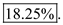c. The cleaning time reduces from 10 minutes to 5 minutes leading to reduction of flow time from 60 minutes to 55 minutes. So, from the Little's law:Since the working time for the restaurant in a typical evening is 4 hours, the number of customers served in an evening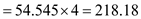So,Other costs remaining the same, the material cost will be as follows:So, the ROIC is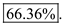d. Keeping all other parameters the same, the overhead changes from \$500 to \$400 per day (the reduction is \$100 per day). So,So,So, the ROIC is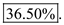The Return-On-Invested Capital (ROIC) for a capital investment scenario can be defined as the following expression:Where, the 'return' is to be computed by deducting all the expenses from the sales for a given period which is generally taken as one year. a. Construct the ROIC tree for the company considering all the information given as follows: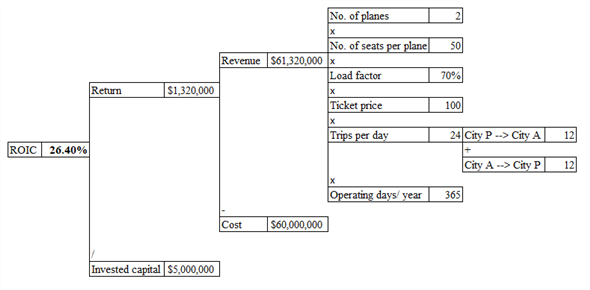b. Compute the ROIC as follows:It is given that the capital invested is \$5,000,000. So,So, the ROIC is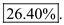c. In order for breaking even, equate the return to zero and compute the value of an unknown load factor L.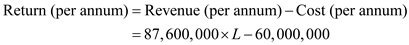Equating the above expression to zero gives: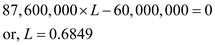So, for the breakeven, the load factor has to be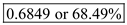d. Let the load factor be L for which the ROIC will plummet from 26.40% to 36.40%.It is given that the capital invested is \$5,000,000. So,Equate the above expression to 36.40% or 0.364 and compute the value of L.So, the load factor required for elevating the ROIC by 10 percent point will be